# Car driver

The car driver is in town A and is scheduled to be in town B at a specified hour. If it travels at an average speed of 50km/h, it will arrive in city B 30 minutes later. But if he travels at an average speed of 70km/h, he would arrive half an hour earlier. Find the distance between cities A and B and the speed at which a driver would have to go to arrive at the specified hour.

s =  175 km
v =  58.3333 km/h

### Step-by-step explanation:

s = 50•(t+30/60)
s = 70•(t-0.50)

60s-3000t = 1500
s-70t = -35

s = 175
t = 3

Our linear equations calculator calculates it.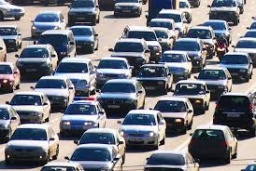Did you find an error or inaccuracy? Feel free to write us. Thank you!Tips to related online calculators
Do you have a linear equation or system of equations and looking for its solution? Or do you have a quadratic equation?
Do you want to convert velocity (speed) units?
Do you want to convert time units like minutes to seconds?

## Related math problems and questions:

• Two citiesThe car goes from city A to city B at an average speed of 70 km/h, back at an average speed of 50 km/h. If it goes to B and back at an average speed of 60 km/h, the whole ride would take 8 minutes less. What is the distance between cities A and B?
• TruckIn 7 hours started from town Krnov truck at speed 40 km/h. Passenger car started against it in 8 hours 30 minutes from the city of Jihlava at speed 70 km/h. Distance between this two cities is 225 km. At what time and at what distance from Krnov this two
• Car and cyclist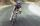Cyclist started from town at a speed of 18 km/h. After 1 hour 30 min started the car from town and caught up with the cyclist in 50 minutes. How fast was a car?
• The carThe car has traveled the distance between A and B for four hour. If we increased the average by 17 km/h the car travel this distance an hour earlier. Determine the initial speed of the car and the distance between A and B.
• Cars speedsDistance from city A to city B is 108 km. Two cars were simultaneously started from both places. The speed of a car coming from city A was 2 km/h higher than the second car. What was the speed of each car if they met in 54 minutes?
• Two cars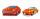From the town A to town B started two cars. The first at 7:00 at average speed 60 km per hour, the second at 10:00 at average speed 100 km per hour. The first car will not stay in B, and on the way back meet the second car at half way from A to B. At what
• Speed of car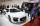The car went to a city that was 240 km away. If his speed increased by 8 km/h, it would reach the finish one hour earlier. Determine its original speed.
• Two trucksTwo trucks left cities A and B against each other and met after an hour. The first car came to B 27 minutes later than the second car to A. Calculate the car speed if the distance between cities A, B is 90 km.
• Two citiesThe distance between cities A and B is 132 km. At 9.00 am, the cyclist started the bike at an average speed of 24 km/h, and at 10.00 am started from the B cyclist at an average speed of 30 km/h. How long and far from A will they both meet?
• DriverThe driver of the supply car reckoned that at the average speed of 72 km/h arrives to the warehouse for 1 1/4 hours. After 30 km however unintentionally drove to the gas station and have ten minutes delay. At what average speed would have to go the rest o
• The cyclist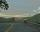The cyclist went from village to town. The first half of the journey went at 20 km/h. The second half of the journey, which mostly fell, went at 39 km/h. All journey took 88 minutes. Calculate the distance from the village to the town.
• Two citiesCities A and B are 200 km away. At 7 o'clock from city A, the car started at an average speed of 80 km/h, and from B at 45 min later the motorcycle is started at an average speed of 120 km/h. How long will they meet and at what distance from the point A i
• Car and motorcyclistA car and a motorcyclist rode against each other from a distance of 190 km. The car drove 10km/h higher than the motorcyclist and started half an hour later. It met a motorcyclist in an hour and thirty minutes. Determine their speeds.
• Train speed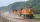The train speed is decreased during 50 sec from 72 km/h to 36 km/h. Assuming that the train movement is equally slowing, find the the acceleration and the distance that it travels at.
• Fog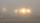The car started in fog at a speed of 30 km/h. After a 12-minute drive, the fog dissipated, and the driver drove the next 12 minutes distance, 17 km. On the last 17 km long again, the driving conditions deteriorated, and the driver drove at a speed of 51 k
• CarThe driver of a car is to get to 608 km distant city. From atlas found that 162 km will have to pass through the cities at average speed 48 km/h. Remainder of the journey pass outside the cities at average speed 116 km/h. Calculate how many hours it will
• Average speedWhen the bus stops at a bus stop driving average speed is 45 km/h. If it did not stop, it would drive at a speed of 54 km/h. How many minutes of every hour does it spend at stops?